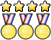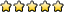Geologic Mapping C

syo_astro
Exalted MemberPosts: 592
Joined: December 3rd, 2011, 9:45 pm
State: NY
Contact:

Re: Geologic Mapping C

The rule says tracing paper and pin. What kind of pin?
The ideal pin would of the capped type sometimes used with nametags. Any type of pin with a flat head should work, provided you can avoid being poked by the tip pointing upwards.
I believe I mentioned an example on the first page: viewtopic.php?f=285&t=12238#p359151 as well as noting that you should find example videos on youtube to see the process (which should help show you why / the type of pin you need): viewtopic.php?f=285&t=12238#p359155. Note for both those cases those are just examples, not necessarily the best or cheapest (aka I was just putting that together quickly as examples, you should look into it yourself of course).

Not sure what Unome means by capped pin for nametags (maybe/probably same thing?), but hopefully that should work/helps. Specify if it doesn't, etc!
B: Crave the Wave, Environmental Chemistry, Robo-Cross, Meteorology, Physical Science Lab, Solar System, DyPlan (E and V), Shock Value
C: Microbe Mission, DyPlan (Earth's Fresh Waters), Fermi Questions, GeoMaps, Gravity Vehicle, Scrambler, Rocks, Astronomy
Grad: Writing Tests/Supervising (NY/MI)

poonicle
MemberPosts: 18
Joined: September 22nd, 2017, 3:11 pm
Division: C

Re: Geologic Mapping C

On one test, the formula for true thickness was given as T = Wsin(d) + Hcos(d) where T = true thickness, W = width, H = height, d = dip, whereas on another test, the formula for true thickness was given as T = Wsin(d) where T = true thickness, W = width, d = dip. Which one is the correct formula? I am very confused.
messed up your nats predicts and what abt it TT____TT

UTF-8 U+6211 U+662F
Exalted MemberPosts: 1518
Joined: January 18th, 2015, 7:42 am
Division: C
State: PA

Re: Geologic Mapping C

On one test, the formula for true thickness was given as T = Wsin(d) + Hcos(d) where T = true thickness, W = width, H = height, d = dip, whereas on another test, the formula for true thickness was given as T = Wsin(d) where T = true thickness, W = width, d = dip. Which one is the correct formula? I am very confused.
What is height?

(Note that a formula for true thickness can be derived using basic trig in emergencies!)

Unome
ModeratorPosts: 4107
Joined: January 26th, 2014, 12:48 pm
State: GA
Location: somewhere in the sciolyverse

Re: Geologic Mapping C

On one test, the formula for true thickness was given as T = Wsin(d) + Hcos(d) where T = true thickness, W = width, H = height, d = dip, whereas on another test, the formula for true thickness was given as T = Wsin(d) where T = true thickness, W = width, d = dip. Which one is the correct formula? I am very confused.
What is height?

(Note that a formula for true thickness can be derived using basic trig in emergencies!)
I would strongly recommend deriving the formula yourself, so you understand how it works. There are several standard formulas for true thickness that cover various situations.
Userpage
Chattahoochee High School Class of 2018
Georgia Tech Class of 2022

Opinions expressed on this site are not official; the only place for official rules changes and FAQs is soinc.org.

Snayhill
MemberPosts: 5
Joined: July 6th, 2018, 9:58 am
Division: C
State: OH

Re: Geologic Mapping C

Hello Everyone!

I was just wondering if anyone here know what lines that are not faults in a relative dating/strata diagram are. Here's a link https://d2vlcm61l7u1fs.cloudfront.net/m ... IiQrsg.png. I'm specifically talking about "G" in this picture.

Thanks!

UTF-8 U+6211 U+662F
Exalted MemberPosts: 1518
Joined: January 18th, 2015, 7:42 am
Division: C
State: PA

Re: Geologic Mapping C

Hello Everyone!

I was just wondering if anyone here know what lines that are not faults in a relative dating/strata diagram are. Here's a link https://d2vlcm61l7u1fs.cloudfront.net/m ... IiQrsg.png. I'm specifically talking about "G" in this picture.

Thanks!
What does line G represent? It's not a fault because there isn't any relative motion across it as you can see in line K

fabishkf
MemberPosts: 13
Joined: February 17th, 2018, 10:41 am
Division: C
State: WI

Re: Geologic Mapping C

joint
Hamilton Middle School '17
Madison West High School '21
National tournaments: 2016 Stout, 2017 Wright State division B, 2018 CSU, 2019 Cornell division C
2019 SONT at Cornell: 2nd Geologic Mapping, 6th Fossils

bluesm0ke
MemberPosts: 1
Joined: January 21st, 2019, 8:07 pm

Re: Geologic Mapping C

On one test, the formula for true thickness was given as T = Wsin(d) + Hcos(d) where T = true thickness, W = width, H = height, d = dip, whereas on another test, the formula for true thickness was given as T = Wsin(d) where T = true thickness, W = width, d = dip. Which one is the correct formula? I am very confused.
What is height?

(Note that a formula for true thickness can be derived using basic trig in emergencies!)
I would strongly recommend deriving the formula yourself, so you understand how it works. There are several standard formulas for true thickness that cover various situations.
Do you think you could post those formulas here? I'm having a hard time grappling more complex true thickness problems that don't just use the basic formula. thanks

Unome
ModeratorPosts: 4107
Joined: January 26th, 2014, 12:48 pm
State: GA
Location: somewhere in the sciolyverse

Re: Geologic Mapping C

What is height?

(Note that a formula for true thickness can be derived using basic trig in emergencies!)
I would strongly recommend deriving the formula yourself, so you understand how it works. There are several standard formulas for true thickness that cover various situations.
Do you think you could post those formulas here? I'm having a hard time grappling more complex true thickness problems that don't just use the basic formula. thanks
I don't have the third one memorized, and can't seem to find it, but I'm pretty sure it's on one of the test exchange tests.
Userpage
Chattahoochee High School Class of 2018
Georgia Tech Class of 2022

Opinions expressed on this site are not official; the only place for official rules changes and FAQs is soinc.org.

poonicle
MemberPosts: 18
Joined: September 22nd, 2017, 3:11 pm
Division: C

Re: Geologic Mapping C

What is height?

(Note that a formula for true thickness can be derived using basic trig in emergencies!)
I would strongly recommend deriving the formula yourself, so you understand how it works. There are several standard formulas for true thickness that cover various situations.
Do you think you could post those formulas here? I'm having a hard time grappling more complex true thickness problems that don't just use the basic formula. thanks
yeah, i'm not gonna lie, i asked that question before TSTs when i didn't know that much about the event. my accuracy improved a lot when i started drawing stuff out then applying trig as opposed to formulas--i'm not very good at identifying which formula applies to which situation yet, but drawing a picture really, really helps imo

that being said, i found T = w*sin(D - S) where D = dip, S = slope, T = true thickness on one practice test. hope that's helpful
messed up your nats predicts and what abt it TT____TT